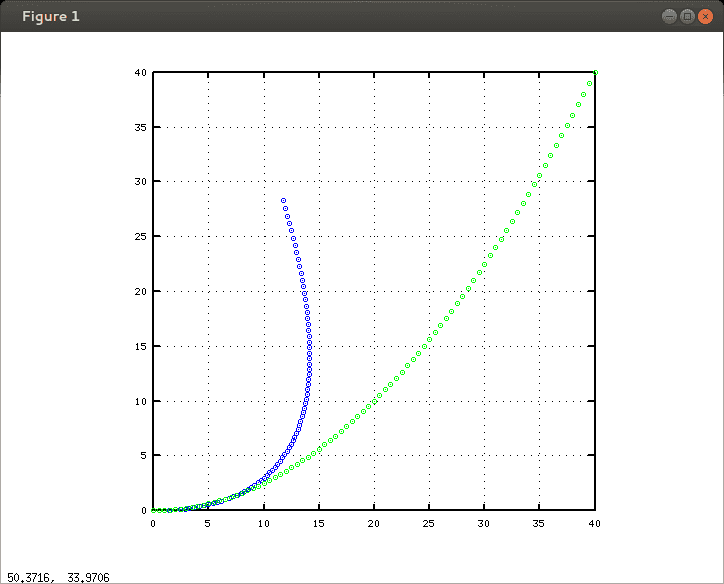# When acceleration and velocity vectors on the same graph.

jlyu002@ucr.e
So basically, on an x-y coordinate system, the acceleration vector is in between 0 degrees and 90 degrees pointing north east, and the velocity component is pointing directly east resting on the x-axis. I was wondering what phenomena this represents in real life and I was also wondering, with the velocity pointing east resting on the x-axis for the next cases, what would it look like when the acceleration is pointing northwest, southwest, and south east. Here are examples of what I know so far. When the acceleration vector is pointing directly west resting on the -x-axis, and when the velocity is pointing directly east resting on the +x-axis the speed of the object is slowing down. The other example is when the acceleration vector is pointing directly north resting on the +y-axis and when the velocity vector is pointing to the east resting on the +x-axis velocity is not increasing and is constant.

For my question I stated above, I know that we can break them apart into vector components i and j; however, I don't understand what that means in real life and what is going on.

Homework Helper
So basically, on an x-y coordinate system, the acceleration vector is in between 0 degrees and 90 degrees pointing north east, and the velocity component is pointing directly east resting on the x-axis. I was wondering what phenomena this represents in real life
Any situation where the applied force is not in the same direction as the velocity: take your pick.
eg. speeding up while going around a corner ... the instantaneous total acceleration will be the sum of centripetal and tangential accelerations ... in this example, the center of the turn is to the north at the instant described. You'd also get this from (say) a hovercraft traveling at a constant speed east while the wind blows from the south-west - pushing it off-course; or where a charged particle is fired at an angle to the electric field.
and I was also wondering, with the velocity pointing east resting on the x-axis for the next cases, what would it look like when the acceleration is pointing northwest, southwest, and south east.
You can work it out by resolving the acceleration vector against the E-W axis ... the N-S component will push it off line and the E-W component will speed it up or slow it down.
Here are examples of what I know so far. When the acceleration vector is pointing directly west resting on the -x-axis, and when the velocity is pointing directly east resting on the +x-axis the speed of the object is slowing down.
Well done!
The other example is when the acceleration vector is pointing directly north resting on the +y-axis and when the velocity vector is pointing to the east resting on the +x-axis velocity is not increasing and is constant.
This is not the case - you have already said that there is an acceleration so, by definition, the velocity cannot be constant.

What happens is that the object moves at the same speed to the East, but gains speed to the North.

Breaking into components is a handy way to analyse the effect of forces/accelerations on motion ... what the force does is change the magnitude and the direction of the momentum vector.

A simple example ... the object moves to the east with constant speed ... then a force is applied producing an acceleration to the west. Initially the object slows down... then it stops ... then it heads off to the west, picking up speed.

If the same force is applied, but always north-west, then the object drifts to the north and slows down, changing direction and speed until it is traveling north-west and picking up speed.

If the same force is applied due north, then the object travels on a parabolic path towards the north... the rate of travel in the easterly direction is not affected.

Homework Helper
Here's an example of two cases...These are the trajectories of two objects both experiencing the same acceleration (0.2cm.s-2) from the same initial velocity (2.0cm.s-1 E) and sampled at 1/4 second intervals over 20 seconds. Both objects start out at the origin. +y is North and +x is East. Axis are labelled in centimeters.

The blue object is accelerating NW while the green one is accelerating due N.

#### Attachments

jlyu002@ucr.e
Woah! Thanks!

jlyu002@ucr.e
Everything else is pretty clear; however, for the situation in which the acceleration points north and the velocity points east, does that mean only direction is changing? Because there is no j component for velocity and an only i component.

jlyu002@ucr.e
oooo i get it. There is an acceleration pointing up. therefore the object traveling in the positive direction is being forced upwards.

jlyu002@ucr.e
Yes! I get it!

Homework Helper
That's right, it's the green one above.

Notice that the distance between circles gets bigger too? This means the total speed is increasing as well as the direction of travel changing.

The blue one has the force 45deg W of N ... as that angle gets closer to 90deg W of N, the curve gets tighter and sharper until, at 90deg W of N = due W, the object just doubles back on itself.

jlyu002@ucr.e
Cool, yeah as the velocity of the object turns into the direction of the acceleration it starts to speed up. Thanks so much Simon. Phew. Lastly, is the graph that you posted position vs time? I think it is because the slope of that graph represents velocity increasing. and the slope velocity increasing would be a constant upward acceleration. Nvm, figured it out. haha.